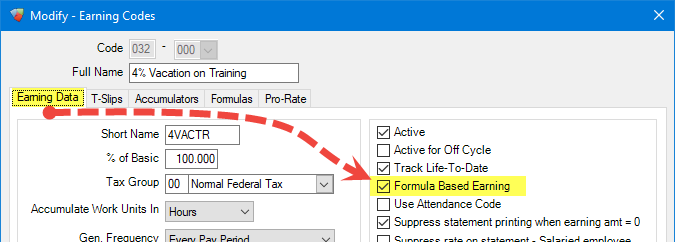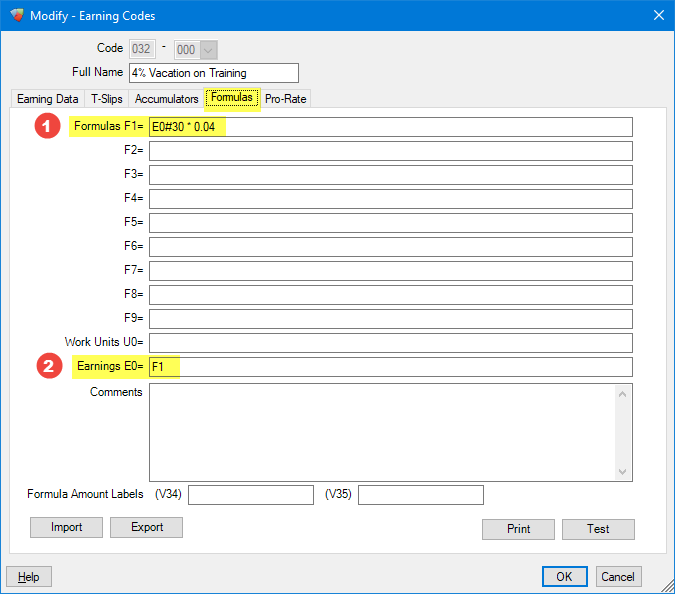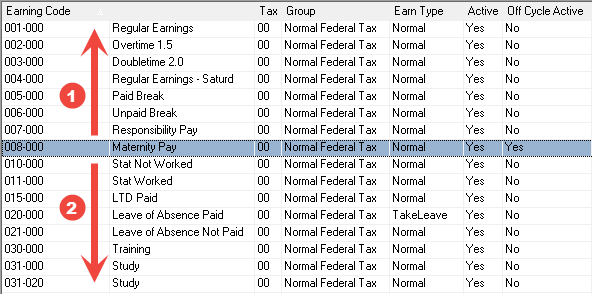# Formulas: Earning Code Formula Basics

Formulas are available within Avanti that enables complex calculations for earnings, deductions, and much more to be performed automatically. Formulas are very powerful and enable different data to be used in the calculations than could normally be accessed. Using a formula with an Earning Code allows other earning code amounts to be referenced when calculating the earnings.

To illustrate how this works we’ll cover how to use a formula with an earning code to generate a 4% vacation amount earning for each hour of Training (another earning code).1 The `Training` Earning Code will be referenced in the formula that we are creating. It is important to note that the Earning Code is `030`.

In order to use a formula on an Earning Code the `Formula Based Earning` option must be enabled from the Earning Data tab of the Earning Code.When this option is enabled the formula tab can be used. Open the formula tab next.

 Formula Variables:The fields F1, F2, through to F9 are Formula Variables. You can enter whatever formulas as needed into these fields and reference the calculated values using the variables names F1, F2,…, F9.Formula variables can contain constant values or complex expression using other variables, math operators, and functions.For example, setting the F1 field to 4 * 3 will return the value 12 whenever the F1 variable is used.1
 F1 formula This formula calculates 4% of the Earnings of Earning Code `30 - Training` by using variable indexing.
2
 Earnings (E0) This value determines how much will be paid for this Earning Code during payroll. The value of the Earnings are assigned using the F1 Formula Variable calculated above.
 Notes:Variable IndexingVariable indexing allows the value for a specific code to be used in a formula.Variables can be indexed by placing a hashtag (#) after the variable and following it with the Earning Code number.For example, indexing variable E0 as E0#30 returns the `Eligible Earnings` for Earning Code `30`PercentagesPercentages cannot be entered directly into formula, and should be entered as decimal amounts.For example 4% is entered as 0.04Math OperatorsBasic math operations can be used in formulas:addition ( +),subtraction ( -),multiplication ( *),division ( /)Different operations can be grouped using parenthesis — ( and ) — to change the order they are evaluated in.

## Earning Code Calculation Order

Earning Code amounts are calculated in ascending order during payroll processing — that means that Earning Code 022 will be calculated before Earning Code 032 during.

 ImportantOur formula above references the Earning Code (030) which happened to be smaller than the formula Earning Code (032). If the formula Earning Code had been 027, 009, or any other code smaller than the Earning Code it was referencing, that value would not be correctly calculated.
• To ensure that the correct value is obtained, the Earning Codes that are referenced within the formula should be lower than the Earning Code containing the formula.

• This means that if the earning code for the formula is 032, the earning codes that are referenced by the formula should all be below 032 (e.g. 017, 031, etc.)

For example, if we are trying to determine the order that Earning Codes will be calculated compared to the Earning Code `008 - Maternity Pay` we can look at the order of the codes.1 All the earning codes with a lower code will be calculated before `Maternity Pay`. 2 All the Earning Codes with a higher code will be calculated after `Maternity Pay`.
 Calculation Step:Alternatively, if you must reference a higher Earning Code from a formula you could adjust the Calculation Step of the Earning Codes.The Avanti system goes through the Earning Codes 9 times in what are called Calc. Steps. You can ensure that the values are correctly calculated by changing the Calc. Step to one that is higher than the referenced Earning Codes.## Generate Automatic Earning

The earnings for the `4% Vacation on Training` Earning Code are going to be automatically generated from any Training earnings of the employee. What this means is that the Earning Code for `4% Vacation on Training` will never be added to a payroll data batch directly, so we need to tell the system that the Earning Code should be still be processed during payroll. This is achieved by enabling one of (or both) of the `Generate automatic earnings` options within the Earning Data tab of the Earning Code.1 Generate automatic earnings - Salaried employeeEnable this option to allow automatic earning of this Earning Code for Salary employees. 2 Generate automatic earnings - Hourly employeeEnable this option to allow automatic earning of this Earning Code for Salary employees.
 Automatic EarningsThe Earning Code must be added to the employee in order for the automatic earnings to generate during payroll.Automatic Earnings allow the Earning Code to generate earnings based on the amount of Training hours that the employee received during the payroll period. If an employee has zero hours of Training in a period, automatic earning will not generate any earnings for the 4% Vacation on Training for that employee.

## Conclusion

The new Earning Code must be added to all employees who should be able to earn this 4% Vacation pay on Training before running a payroll.

When the employee works a certain number of hours of Training (Earning Code 030) the 4% Vacation on Training (Earning Code 032) will automatically generate a 4% earnings from the total Training earnings on the payroll.

For example, here an employee was paid \$100 of Training during a period, and received \$4 of Vacation on Training earnings on their payroll.Did you find it helpful? Yes No

Send feedback